# Quiz 14: Information Security and Computer Fraud

Organizations today are growing in scale and have made improvement in their processes, practices of the project management. It assesses their current practices compared to the recognized best practices of the industry. It also helps in focusing on opportunities which can initiate developments in the performance and the results too at business level. But there are various other IT projects for which alternatives available to implement. Hence, they need to be evaluated before their implementation. The series of questions generally upsurge while making an IT investment which can be depicted as: 1. What is the purpose of that project? 2. Which business issues will be covered by this project? 3. What will be the cost of this project (as the project needs to be at the limit of finance available in the firm)? 4. What is the return of that project and possible associated risks to it. It implies that the return should be more than initial investment and minimum risks should be present. 5. Are there any alternatives available in respect to the project, which requires less finance or heavy returns? 6. If the project had implemented, what techniques will be required to measure its success? After getting answers of above all questions only decision is required to be taken. Option a is correct as cost is the important part of project implementation as specified above. Option b is correct as associated risks to project are the important part of the project implementation as specified above. Option c is correct as alternatives are the important part of project implementation as specified above. Option d is correct as the evaluation of success is the important part of project implementation as specified above. The option e indicates that all the options (costs, related risks, available alternatives, techniques for success evaluation) is correct because firms require minimum investment and maximum return from any large IT project. Thus, the option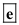is correct.
Pay Back Period: It is the time period required to cover the cost of Investment. It is relevant for decision making to Investors. Net Present Value (NPV): It is the difference between the present value (PV) of cash inflows and present value (PV) of cash outflows of the project and it is relevant for decision making. Internal Rate of Return (IRR): It is that rate of return under which, the present value (PV) of cash inflow is equals to the present value (PV) of cash outflow. In other words, the net present value (NPV) of the Investment becomes nil. Accounting Rate of Return (ARR): Accounting rate of return means the return earned by an investor in the proposed project. Under this method the time value of money is not considered. a. Company ABC has following information without considering the risk factors and it is presented in the table using Excel sheet is given below: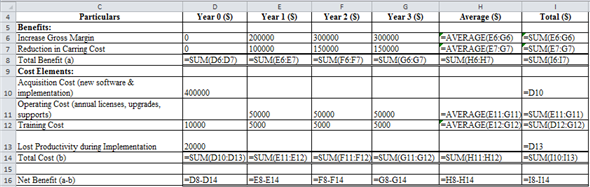The result of the above Excel sheet is given below: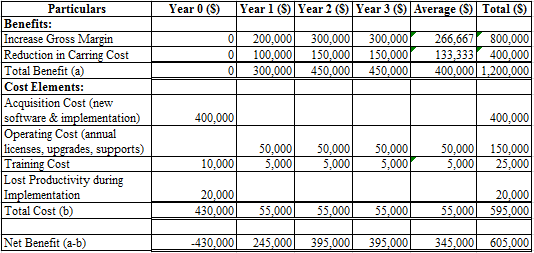Net benefit from the project is calculated through the deduction of cost element from the benefits earned in the project. From the information given above it is required the following: (1) Calculate the Payback period of the project:Hence, the payback period of the project is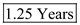(2) Calculate the Net Present Value (NPV) of the project. Following is the calculation of NPV using the Excel sheet:The result of the above Excel sheet is given below: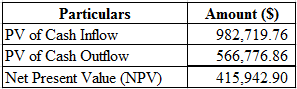Hence, the Net Present Value (NPV) of the project is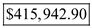(3) Calculate the Internal rate of return (IRR) of the project. Following is the calculation of IRR using the Excel sheet: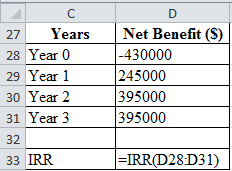The result of the above Excel sheet is given below: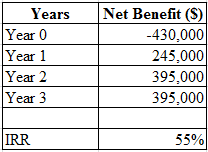Hence, the IRR of the project is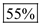(4) Calculate the Accounting rate of return (ARR) of the project.Hence, the ARR of the project is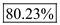b. The following information after considering risk factors is presented in the table given below using Excel sheet: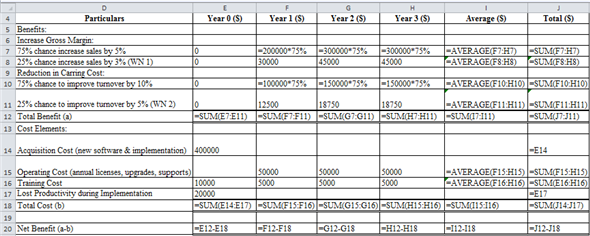The result of the above Excel sheet is given below: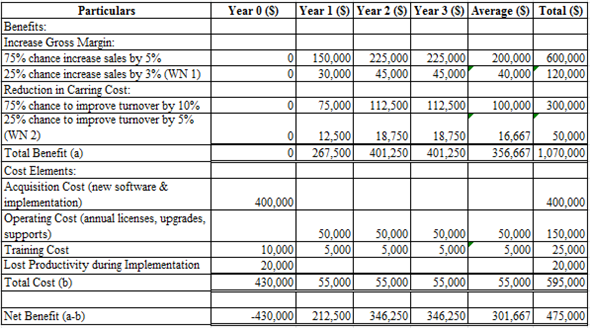Net benefit from the project is calculated through the deduction of cost element from the benefits earned in the project. Working Note 1: Following is the calculation of Increase in gross margin when sale is increase by 3% using Excel sheet: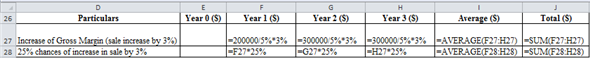The result of the above Excel sheet is given below: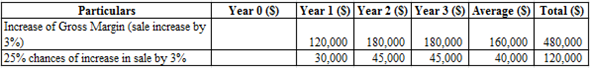Working Note 2: Following is the calculation of reduction in carrying cost when inventory turnover is increase by 5% using Excel sheet: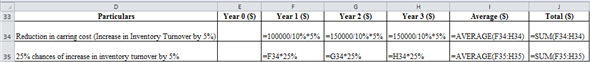The result of the above Excel sheet is given below: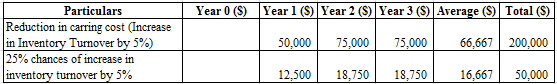(1) Calculate the Payback period of the project:Hence, the payback period of the project is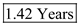(2) Calculate the Net Present Value (NPV) of the project. Following is the calculation of NPV using the Excel sheet:The result of the above Excel sheet is given below: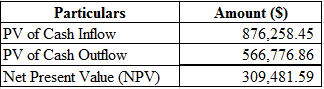Hence, the Net Present Value (NPV) of the project is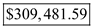(3) Calculate the Internal rate of return (IRR) of the project. Following is the calculation of IRR using the Excel sheet: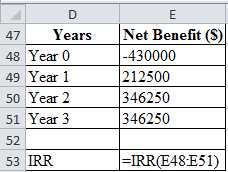The result of the above Excel sheet is given below: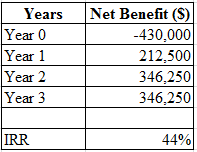Hence, the IRR of the project is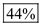(4) Calculate the Accounting rate of return (ARR) of the project.Hence, the ARR of the project is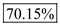c. Value proposition is the final step which assembles the cost, benefits and risks of the project outlined above. Based on the above financial measures and risk analysis, company should pursue the project. However, this analysis has not considered whether company's employees will be willing to change (change risk) or whether this investment is consistent with company's strategy (alignment risk). Additionally the sensitivity analysis is limited.